Courses

# Previous Year Questions - Electricity Class 10 Notes | EduRev

## Class 10 : Previous Year Questions - Electricity Class 10 Notes | EduRev

The document Previous Year Questions - Electricity Class 10 Notes | EduRev is a part of the Class 10 Course Science Class 10.
All you need of Class 10 at this link: Class 10

Q.1. On what factors does the resistance of a conductor depend ?
Or
List the factors on which the resistance of a conductor in the shape of a wire depends.   [CBSE 2019]
Ans:
Resistance of a conductor:
(i) is directly proportional to its length
(ii) inversely proportional to its cross-section area and depends on the material of the conductor.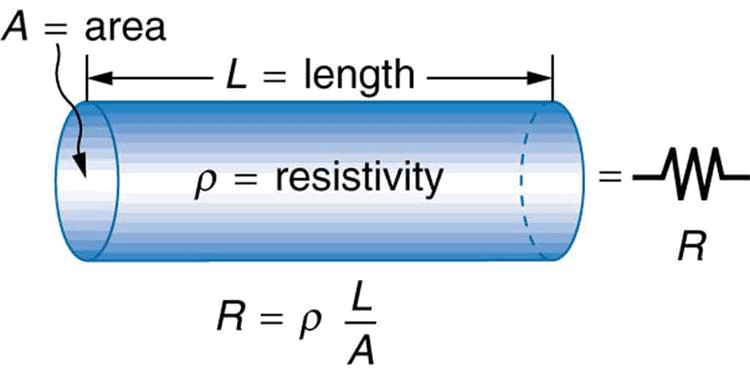Resistance also depends on the temperature.

Q.2. (a) A bulb is rated 40 W, 220 V. Find the current drawn by it when it is connected to a 220 V supply. Also find its resistance.
(b) If the given blub is replaced by a blub of rating 25 W, 220 V, will there be any change in the value of current and resistance? Justify your answer and determine the change.   [CBSE 2019]

Ans: (a) Here power of bulb P = 40 W and voltage V = 220 V
∴ Current drawn by the bulb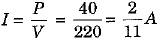and resistance of the bulb R =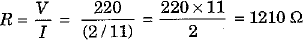(b) On taking another bulb of power P' = 25 W and voltage V = 220 V, there is a change in the value of current and resistance because their values depend on the power of the bulb.
New current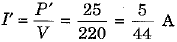and new resistance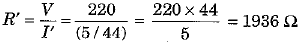Q.3. Derive the expression for power P consumed by a device having resistance jR and potential difference V.
Or
A device of resistance R is connected across a source of V voltage and draws a current I . Derive an expression for power in terms of voltage (or current) and resistance.   [CBSE 2019]
Ans:
Amount of work done in carrying a charge Q through a potential difference V is
W = QV.
But Q = It  ∴ W = VIt
As power is defined as the rate of doing work, hence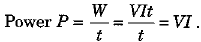If R be the value of resistance of the conductor, then V - RI and hence
P = VI = (RI)I = I2R
Again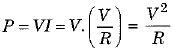Thus, in general we can say that electric power is given by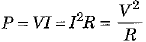Q.4. Compare the power used in 2 Ω resistor in each of the following circuits shown in Fig. 12.36 (a) and (b) respectively.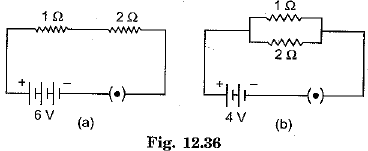Ans: When a 2 Ω resistor is joined to a 6 V battery in series with 1 Ω and 2 Ω resistors, total resistance of the combination Rs = 2 + 1 + 2 = 5Ω
∴ Current in the circuit I=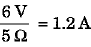∴ Power used in the 2Ω resistor P=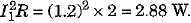(ii) When 2 Ω resistor is joined to a 4 V battery in parallel with 12 Ω and 2 Ω resistors, current flowing in 2 Ω resistor is independent of the other resistors.
∴ Current flowing through 2Ω resistor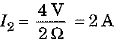∴ Power used in the 2Ω resistor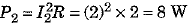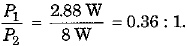Q.5. Derive the relation R = R1 + R2 + R3 when resistors are joined in series.   [CBSE 2019]
Ans: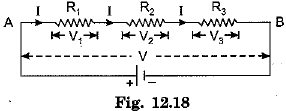In series combination, the same current flows in all the resistances but the potential difference across each of the resistance is different.
According to Ohm ’s law, we have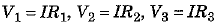If the total potential difference between A and B is V, then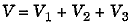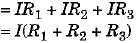Let the equivalent resistance be R, then
V = IR
and hence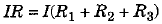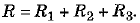Q.6. When do we say that the potential difference between two points of a circuit in 1 volt ?   [CBSE 2019]
Ans:
Potential difference between two points of an electric circuit is said to be 1 volt, when a work of 1 J is to be done for moving a charge of 1 C between these two points.

Q.7. Define resistance. Give its SI unit.   [CBSE 2019]
Ans.
Resistance of a conductor is the measure of opposition offered by it for the flow of electric charge through it. SI unit of resistance is ‘ohm’ (Ω).

Q.8. State ohm’s law.   [CBSE 2019]
Ans
: According to Ohm’s law, temperature remaining constant, the current passing through a conductor is directly proportional to the potential difference across its ends, i.e.,
V ∝ I or V = IR
Here, constant R is known as the resistance of given conductor.

Q.9. (a) Write the relation between resistance and electrical resistivity of the material of a conductor in the shape of a cylinder of length 'l' and area of crosssection 'A' Hence derive the SI unit of electrical resistivity.
(b) Resistance of a metal wire of length 5 m is 100 Ω. If the area of crosssection of the wire is 3 x 10-7 m2, calculate the resistivity of the material.   [CBSE 2019]

Ans: (a) The resistance R of a conductor, the shape of a cylinder, of length l and area of cross-section A is given as: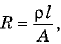where ρ is a constant, which is known as the electrical resistivity of the material of conductor.
Thus, resistivity ρ = RA/l
∴ SI unit of resistivity ρ shall be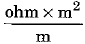=  Ω-m
(b) Here l = 5 m, R = 100 Ω and A = 3 x 10-7 m2
∴ Resistivity of the material of wire ρ = RA/l =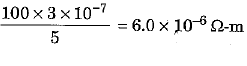Q.10. (a) With the help of a suitable circuit diagram prove that the reciprocal of the equivalent resistance of a group of resistors joined in parallel is equal to the sum of the reciprocals of the individual resistances.
(b) In an electric circuit two resistors of 12Ω each are joined in parallel to a 6 V battery. Find the current drawn from the battery.   [CBSE 2019]

Ans: (a)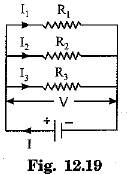In parallel combination of three resistances R1 R2 and R3, the current in each of the resistances is different. If I is the current drawn from the cell then it is divided into branches I1 Iand I3. Thus,
I = I1 + I2 + I3.
The potential difference across each of these resistances is the same.
Thus, from Ohm’s law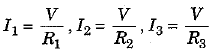If R is the equivalent resistance then,
I = V/R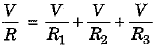and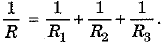(b) Here R1 = R2 = 12 Ω and V = 6 V
Net resistance R o f the parallel grouping is :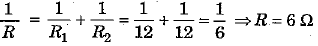∴ Current drawn by the circuit from the battery I =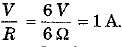Q.11. Study the circuit of Fig. 12.24 and find out : (i) Current in 12 Ω, resistor (ii) difference in the readings of A1 and A2 if any. [CBSE 2019]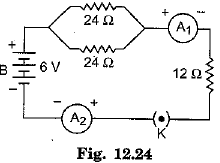Ans: (i) In the circuit resistor of R1 = 12 Ω is connected in series with parallel combination of two resistors R2 and R3 of 24 Ω each.
The effective resistance of parallel combination of R2 and R3 is given as :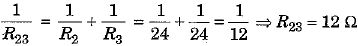∴ Net resistance of the circuit R = R1 + R23 = 12 + 12 = 24 Ω
∴ Current through 12 Ω resistor = Circuit current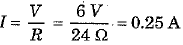(ii) Both ammeters A1 and A2 give same reading of 0.25 A and there is no difference in their readings.

Q.12. (i) Draw a schematic diagram of a circuit consisting of a battery of five 2 V cells, a 5 Ω resistor, a 10 Ω  resistor and a 15 Ω  resistor and a plug key all connected in series.
(ii) Calculate the electric current passing through the above circuit w hen the key is closed. (iii) Potential difference across 15 Ω  resistor. [CBSE 2019]

Ans: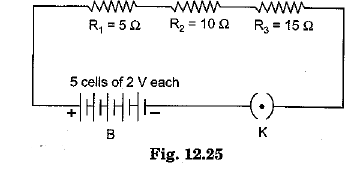(i) The schematic diagram is given in Fig. 12.25.
(ii) Here total voltage V = 5 x 2 = 10 V
and total resistance R = R1 + R2 + R3
= 5 + 10 + 15 = 30 Ω
∴ Current passing through the circuit when the key is closed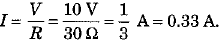(iii) Potential difference across resistor R3 of 15 Ω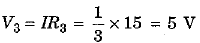Q.13. Show how would you join three resistors, each of resistance 9 Ω so that the equivalent resistance of the combination is (i) 13.5 Ω, (ii) 6 Ω ?   [CBSE 2018]
Ans: Here resistances R1 - R2 = R3 = 9Ω
(i) To obtain an equivalent resistance Req = 13.5Ω, we connect one resistor R1 in series to the parallel com bination o f R2 and R3 as shown in figure (i). Then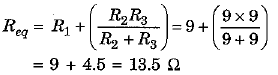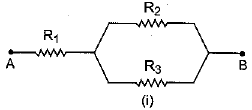(ii) To obtain equivalent resistance Req = 6 Ω, we connect resistor Rin parallel to the series combination of R2 and R3 as shown in figure (ii). Then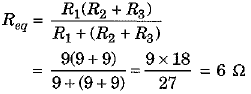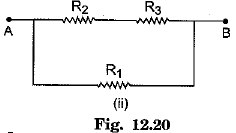Q.14. State Joule’s law of heating.   [CBSE 2018]
Ans:
A s per Joule’s law the heat produced in a resistor is (i) directly proportional to square of current flowing through it, (ii) directly proportional to resistance, and (iii) directly proportional to time. Mathematically,
Heat H = I2Rt

Q.15. Why are metals good conductors of electricity whereas glass is a bad conductor of electricity ? Give reason. [CBSE 2018]
Ans:
Metals are good conductors of electricity because they have a large number of free (conduction) electrons which can easily conduct electricity. On the other hand, glass has no free electrons. Thus conduction of charge is not easily possible in glass and so glass is a bad conductor of electricity.

Q.16. Nichrome is used to make the element of an electric heater. Why ? [CBSE 2017]
Ans: Nichrome is an alloy of high resistivity and high melting point and does not oxidise easily.

Q.17. Derive the relation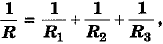when resistors are joined in parallel. [CBSE 2017]
Ans: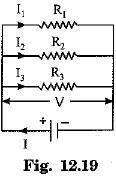In parallel combination of three resistances R1 R2 and R3, the current in each of the resistances is different. If I is the current drawn from the cell then it is divided into branches I1 Iand I3. Thus,
I = I1 + I2 + I3.
The potential difference across each of these resistances is the same.
Thus, from Ohm’s lawIf R is the equivalent resistance then,
I = V/RandQ.18. Why do electricians wear rubber hand gloves while working ?  [CBSE 2016]
Ans:
Rubber is an electrical insulator. Hence electrician can work safely while working on an electric circuit without a risk of getting any electric shock.

Q.19. How are two resistors with resistances R1Ω and R2Ω are to be connected to a battery of emf 3 volts to obtain maximum current flowing through it ?   [CBSE 2016]
Ans:
For maximum current flow, the net resistance of circuit must be least possible. Hence resistors R1 and R2 should be connected in parallel.

Q.20. What potential difference is needed to send a current of 5 A through the electrical appliance having a resistance of 18 Ω ?   [CBSE 2016]
Ans: Potential difference V = RI - 18 x 5 = 90 V.

Q.21. Power of a lamp is 60 W. Find the energy in SI unit consumed by it in 1 s.   [CBSE 2016]
Ans: Energy consumed E = Pt = 1 W x 1 s - l J .

Q.22. (a) What do you mean by resistance of a conductor ? Define its unit.
(b) In an electric circuit with a resistance wire and a cell, the current flowing is I . What would happen to this current if the wire is replaced by another thicker wire of same material and same length ? Give reason. [CBSE 2016]
Ans. (a) The resistance of a conductor is a property of the conductor, which affects the flow of current through it on maintaining a potential difference across its ends.
Unit of resistance is ohm. Resistance of a conductor is said to be 1 ohm, if a potential difference of 1 V is to be applied across its ends for m aintaining flow of 1 A current.
(b) If given resistance wire is replaced by another thicker wire o f same material and same length then cross-section area of wire is increased and consequently its resistance decreases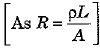So the current flowing in the circuit increases.

Q.23. (a) Why are electric bulbs filled with chemically inactive nitrogen or argon gas?
(b) The resistance of a wire of 0.01 cm radius is 10 Ω. If the resistivity of the material of the wire is 50 x 10-8 Ω-m, find the length of the wire. [CBSE 2016]
Ans: (a) Electric bulbs are generally filled with some inert gas like nitrogen or argon. This enables to prolong the life of the filament of electric bulb.
(b) Here radius of wire r = 0.01 cm = 0.01 x 10-2 m, resistance R = 10 Ω and resistivity p = 50 x 10-8 Ω-m.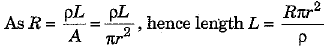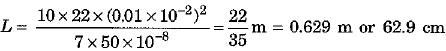Q.24. (a) Define electric power. Express it in terms of V, I and R where V stands for potential difference, R for resistance and I for current.
(b) V -I graphs for two wires A and B are shown in the Fig. 12.34. Both of them are connected in series to a battery. Which of the two will produce more heat per unit time ? Give justification for your answer. [CBSE 2016]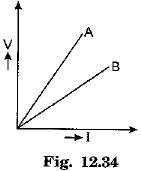Ans: (a) Electric power is defined as the rate of supplying electrical energy for maintaining current flow through a circuit.
Electric power P = VI = I2R = V2/R.
(b) We know that slope of V-I graph for a given wire gives its resistance and in given figure slope of graph is more for wire A. It means that RA > RB.
In series arrangement same current I flows through both the resistance.
As heat produced per unit time is given by I2R, hence it is obvious that more heat will be produced per unit time in wire A.

Q.25. Identify the following symbols of commonly used components in a circuit diagram:   [CBSE 2015]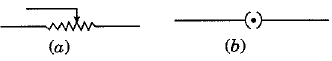Ans. (a) A rheostat, (b) A closed plug key.

Q.26. Define electric current.   [CBSE 2015]
Ans:
Time rate of flow of charge through any cross-section of a conductor is called electric current.

Q.27. State the SI unit of electric current and define it.   [CBSE 2015]
Ans:
An ampere (A), which is defined as the rate of flow of 1 coulomb of charge per second.

Q.28. In an electric circuit, state the relationship between the direction of conventional current and the direction of flow of electrons.   [CBSE 2015]
Ans:
In an electric circuit, the direction of conventional current is taken as opposite to the direction of flow of electrons.

Q.29. What is meant by the statement "potential difference between points A and B in an electric field is 1 volt” ?   [CBSE 2015]
Ans:
Amount of work done to bring 1 C charge from point B to point A in the electric field is 1 joule.

Q.30. Define kW h. [CBSE 2015]
Ans. A kilowatt hour (kW h) is the commercial unit of electrical energy. It is the energy consumed when 1 kW (1000 W) power is used for 1 hour.

Q.31. How many joules are equals to 1 kW h ? [CBSE 2015]

Ans: 3.6 x 106 J = 1 kW h.

Q.32. Define an electric circuit. Draw a labelled, schematic diagram of an electric circuit comprising of a cell, a resistor, an ammeter, a voltmeter and a closed switch. Distinguish between an open and a closed circuit. [CBSE 2015]
Ans: A continuous and closed path of an electric current is called an electric circuit.
A labelled, schematic diagram of an electric circuit showing a cell E, a resistor R, an ammeter A, a voltmeter V and a closed switch S is shown here.
An electric circuit is said to be an open circuit when the switch is in ‘off’ mode (or key is unplugged) and no current flows in the circuit.
The circuit is said to be a closed circuit when the switch is in 'on' mode (or key is plugged) and a current flows in the circuit.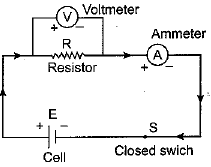Q.33. (a) n electrons, each carrying a charge -e, are flowing across a unit crosssection of a metallic wire in unit time from east to west. Write an expression for electric current and also give its direction of flow. Give reason for your answer.
(b) The charge possessed by an electron is 1.6 x 10-19 coulomb. Find the number of electrons that will flow per second to constitute a current of 1 ampere. [CBSE 2015]
Ans: (a) Electric current in a circuit is defined as the time rate of flow of electric charge through any cross-section and its direction is opposite to that of flow of electrons. Hence in present case
electric current I =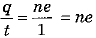As electrons are flowing from east to west, the direction of electric current is from west to east.
(b) Here current I = 1 A, time t = 1 s and charge on each electron e = 1.6 x 10-19 C.
Hence, number of electrons flowing n =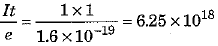Q.34. (a) List the factors on which the resistance of a cylindrical conductor depends and hence write an expression for its resistance.
(b) How will the resistivity of a conductor change when its length is tripled by stretching it ? [CBSE 2015]

Ans. (a) The resistance of a cylindrical conductor i.e., a wire (R) is (i) directly proportional to its length L, (ii) inversely proportional to its cross-section area A and (iii) depends on the nature of material of wire. Mathematically,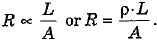Here, ρ is known as the resistivity of given material. It is defined as the resistance offered by a unit cube of given material when current flows perpendicular to the opposite faces. (b) The resistivity of the conductor remains unchanged.

Q.35. (a) V-I graphs for two wires A and B are shown in the Fig. 12.17. If both the wires are made of same material and are of same length, which of the two is thicker? Give justification for your answer.
(b) A wire of length L and resistance R is stretched so that the length is doubled and area of cross-section halved.

How will (i) resistance change, and (ii) resistivity change ? [CBSE  2015]Ans. (a) Resistance R of wire A is more than that of B (RA > RB) because slope of V-I graph for A is more. The resistance of a wire is inversely proportional to its cross-section area. Hence area of cross-section of wire B, of smaller resistance, must be more. Thus, wire B is thicker.
(b) (i) The new resistance of wire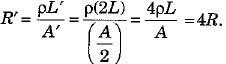Thus, resistance increases to four times its original value.
(ii) The resistivity remains unchanged because it does not depend on the dimensions of a conductor of given metarial.

Q.36. Study the electric circuit of Fig. 12.21 and find (i) the current flowing in the circuit, and (ii) the potential difference across 10 Ω resistor. [CBSE 2015]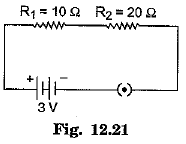Ans. (i) Here V = 3 V, R1 = 10 Ω and R 2 = 20 Ω. Since R1 and R2 are connected in series, the effective resistance of the circuit
R = R1 + R2 = 10 + 20 - 30 Ω
∴ Current flowing in the circuit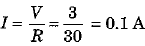(ii) The potential difference across R 1 = 10 Ω resistor is V1 = IR1 = 0.1 x 10 = 1.0 V.

Q.37. Three resistors of 3 Ω each are connected to a battery of 3 V as shown in Fig. 12.22. Calculate the current drawn from the battery. [CBSE 2015]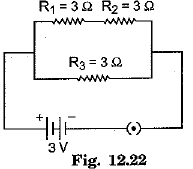Ans: In the electric circuit shown the series combination of R1 and R2 is joined in parallel to the resistance R3. Hence equivalent resistance R of the circuit is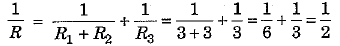⇒ R = 2 Ω
∴ Current drawn from the battery I=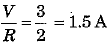Q.38. Find the equivalent resistance across the two ends A and B of the circuit [Fig. 12.31]. [CBSE 2015]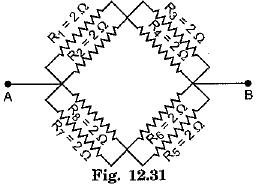Ans: Equivalent resistance of R1 and joined in parallel is R2 , where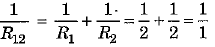⇒ R12 = 1Ω
Similarly R34 = R56 = R78 = 1Ω
Now R12 and R34 are in series making a resistance
Rq = R12 + -R34 = 1 + 1 = 2Ω
Similarly R56 and R78 are in series and make a resistance R10 = 2Ω
Finally R9 and R10 are connected in parallel between the points A and B and hence equivalent resistance R is given as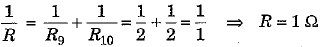Q.39. A 5 Ω resistor is connected across a battery of 6 volts. Calculate :
(i) the current flowing through the resistor.
(ii) the energy that dissipates as heat in 10 s. [CBSE 2015]

Ans: Here V = 6 V and R = 5 Ω
(i) The current flowing through the resistor I =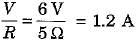(ii) Energy dissipated as heat in time t = 10 s is
∴ H = I2Rt = (1.2)2 x 5 x 10 = 72 J

Q.40. Calculate the amount of heat generated while transferring 90000 coulombs of charge between the two terminals of a battery of 40 V in one hour. Also determine the power expended in the process. [CBSE 2015]
Ans: Here charge transferred Q = 90000 C, potential difference b etw een the terminals o f battery V = 40 V and time t = 1 h = 3600 s.
Current =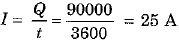Amount of heat generated H = Vlt = 40 x 25 x 3600 = 3600000 J = 3.6 x 106 J
and power expended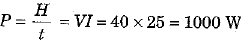Q.41. How many 40 W; 220 V lamps can be safely connected to a 220 V, 5 A line ?
Ans. The current drawn by a 40 W, 220 V electric lamp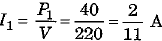As the electric line is o f rating 220 V, 5 A, hence we can connect n lamps in parallel where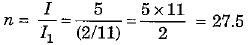Thus, we can safely connect 27 lamps of 40 W, 220 V rating to a 220 V, 5 A line.

Q.1. An electric lamp of resistance 20 Ω and a conductor of resistance 4 Ω are connected to a 6 V battery as shown in the circuit. Calculate
(а) the total resistance of the circuit,
(b) the current through the circuit,
(c) the potential difference across the (i) electric, lamp and (ii) conductor, and
(d) power of the lamp.   [CBSE 2019]
Ans:
Here voltage of battery V = 6 V, resistance of electric lamp = R= 20 Ω  and resistance of conductor R2 = 4 Ω.
(a) Since R1 and R2 are connected in series, the total resistance of the circuit
R = R1 + R2 = 20 + 4 = 24 Ω
(b) The current through the circuit I = V/R = 6/24 = 0.25 A
(c) (i) Potential difference across the electric lamp V1 = IR= 0.25 x 20 = 5 V
(ii) Potential difference across the conductor V= IR= 0.25 x 4 = 1 V
(d) Power of the lamp P = I2R1 = (0.25) x 20 = 1.25 W

Q.2. (a ) Three resistors R1, R2 and R3 are connected in parallel and the combination is connected to a battery, ammeter, voltmeter and key. Draw suitable circuit diagram and obtain an expression for the equivalent resistance of the combination of the resistors.
(b) Calculate the equivalent resistance of the network shown in Fig. 12.38 [CBSE 2019]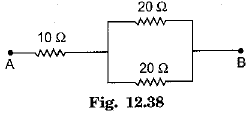Ans: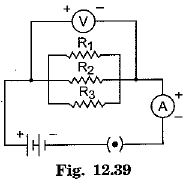(a) The arrangement is shown in circuit diagram of Fig. 12.39.
In parallel combination of three resistances R1 R2 and R3, the current in each of the resistances is different. If I is the current drawn from the cell then it is divided into branches I1 Iand I3. Thus,
I = I1 + I2 + I3.
The potential difference across each of these resistances is the same.
Thus, from Ohm’s lawIf R is the equivalent resistance then,
I = V/Rand(b) In the network resistors of 20 Ω and 20 Ω are joined in parallel and make a resistance R1, where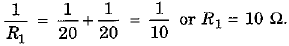This combined resistance R1 = 10 Ω is joined in series with given 10 Ω resistance. Hence equivalent resistance of the network will be R = 10 + 10 = 20 Ω.

Q.3. (a) Three resistors o f resistances R1, R2 and R3 are connected in (i) series, and (ii) parallel. Write expression for the equivalent resistance of the combination in each case.
(b) Two identical resistances of 12 Ω each are connected to a battery of 3 V.

Calculate the ratio of the power consumed by the resulting combinations with minimum resistance and maximum resistance. [CBSE 2019]
Ans: (a) (i) In series arrangement, equivalent resistance Rs = R1 + R2 + R3
(ii) In parallel arrangement, equivalent resistance Rp is given as: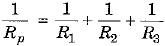(b) Here = R1 = R= 12 Ω and V = 3V.
For minimum resistance, two resistors must be connected in parallel so that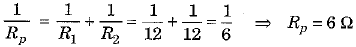Hence power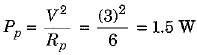For maximum resistance, two resistors must be connected in series so that
Rs = R1 + R2 = 12 + 12 = 24 Ω
So, the power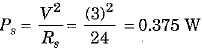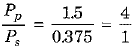Q.4. Experimentally prove that in series combination of three resistances :
(а) current flowing through each resistance is same, and
(b) total potential difference is equal to the stun of potential differences across individual resistors. [CBSE 2019]

Ans: Series combination o f resistors : We take three resistors R1 R2 and R3 and join them in series between the points X and Y in an electric circuit as shown in Fig. 12.42.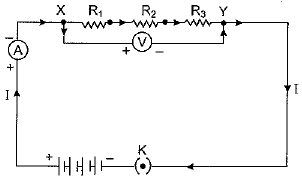(a) Plug the key and note the ammeter reading.
Then change the position of ammeter to anywhere in between the resistors and again note the ammeter reading. We find that ammeter reading remains unchanged. It shows that in series arrangement same current flows through each resistor.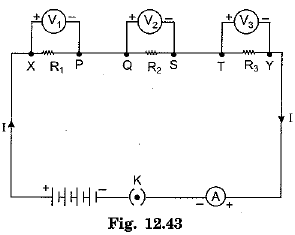(b) Insert a voltmeter across the ends X and Y of the series combination of resistors. Plug the key so as to complete the circuit and note the voltmeter reading V across the series combination of resistors.
Take out plug from key K and disconnect the voltmeter. Now insert the voltmeter across the ends of first resistor R1 as shown in Fig. 12.43. Plug the key and note the voltmeter reading V1. Similarly, measure the potential difference across the other two resistors R2 and R3 separately. Let these potential differences be V2 and V3, respectively. Experimentally we find that
V = V1 + V2  + V3
It shows that in series arrangement of resistors total potential difference is equal to the sum of potential differences across individual resistors.

Q.5. (a) Establish a relationship to determine the equivalent resistance IS of a combination of three resistors having resistances R1, R2 and R3 connected in parallel.
(b) Three resistors are connected in an electrical circuit as shown. Calculate the resistance between A and B. [CBSE 2016]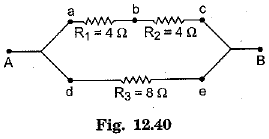Ans:
(a) The arrangement is shown in circuit diagram of Fig. 12.39.
In parallel combination of three resistances R1 R2 and R3, the current in each of the resistances is different. If I is the current drawn from the cell then it is divided into branches I1 Iand I3. Thus,
I = I1 + I2 + I3.
The potential difference across each of these resistances is the same.
Thus, from Ohm’s lawIf R is the equivalent resistance then,
I = V/Rand(b) Here series combination of R1 and R2 is joined to R3 in parallel arrangement. Hence the net resistance R between points A and B is given as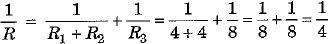⇒ r = 4 Ω

Q.6. (a) Establish a relationship to determine the equivalent resistance R of a combination of three resistors having resistances R1, R2 and R3 connected in series.
(b) Calculate the equivalent resistance R of a combination of three resistors of 2 Ω, 3 Ω and 6 Ω joined in parallel. [CBSE 2016]

Ans: (a)In series combination, the same current flows in all the resistances but the potential difference across each of the resistance is different.
According to Ohm ’s law, we haveIf the total potential difference between A and B is V, thenLet the equivalent resistance be R, then
V = IR
and hence(b) Here = R= 2 Ω R2 = 3 Ω and R3 = 6 Ω
The equivalent resistance R for parallel combination of resistors will be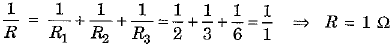Q.7. What is meant by electric current ? Name and define its SI unit. In a conductor electrons are flowing from B to A. What is the direction of conventional current ? Give justification for your answer.
A steady current of 1 ampere flows through a conductor. Calculate the number of electrons that flow through any section of the conductor in 1 second.
(Charge on electron — 1.6 x 10-19 coulomb) [CBSE 2015]
Ans. Electric current is defined as the rate of flow of electric charge through a cross- section of a conductor. If Q charge passes through a section of a conductor in time t, then current I = Q/t.
SI unit of electric current is an ampere (A). Current is said to be one ampere, if rate of flow of charge through a cross-section of conductor be 1 coulomb per second.
Direction of conventional current is taken as the direction of flow of positive charge or opposite to the direction of flow of negative charge. If negatively charged in a conductor flow from B to A then the direction of conventional current will be from A to B.
Here current I = 1 A, time t = 1 s and charge on electron e = 1.6 x 10-19 C. Let n electrons flow through a section of conductor so that charge passing through the section is Q = ne.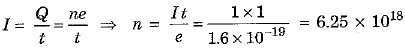Q.8. What is heating effect of electric current ? Find an expression for amount of heat produced. Name some appliances based on heating effect of current. [CBSE 2015]
Ans: When a current flows through a conducting wire (resistance wire), heat is developed and temperature of wire rises. It is known as the heating effect of electric current.
If V be the potential difference maintained across the ends of a wire then, by definition, the amount of work done for flow of 1 C charge through the wire is V.
∴ Work done for flow of Q charge
W = VQ = VIt  [∵ Q = It]
where I is the current flowing in time t.
As V = IR, hence
W= VIt = (IR)It = I2Rt
This work done (i.e., electrical energy dissipated) is converted into heat. Hence, the amount of heat produced,
Q = I2Rt J
This is known as Joule’s law of heating.
Incandescent lamps, electric iron, electric stove, toaster, geyser, electric room heater etc., are the appliances based on heating effect of electric current.

Q.9. The values of current J flowing in a given resistor for the corresponding values of potential difference V across the resistor are given below: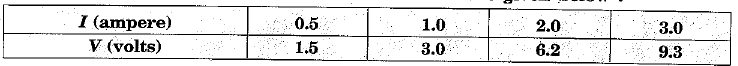(i) Plot a graph between V and I.
(ii) Calculate the resistance of that resistor.
(iii) What does the graph represent ?   [CBSE 2015]

Ans:(i) The plotted V-I graph is shown in Fig. 12.37.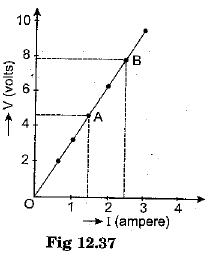(ii) We take two points A and B on the graph for which currents are 1.5 A and 2.5 A respectively and the corresponding values of V are 4.6 V and 7.8 V.

∴ Resistance of resistor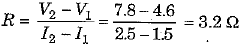(iii) The V-I graph is linear one. It shows that the given resistor strictly obeys Ohm’s law.

Offer running on EduRev: Apply code STAYHOME200 to get INR 200 off on our premium plan EduRev Infinity!

## Science Class 10

80 videos|266 docs|162 tests

,

,

,

,

,

,

,

,

,

,

,

,

,

,

,

,

,

,

,

,

,

;